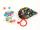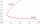# Legs

In the room are four-legged chairs, three-legged stool, and all are sitted with (one) people. I counted all the leg room and there were a total of 39. How many are there chairs, stool and people?

Result

x =  4
y =  3
z =  7

#### Solution:Leave us a comment of example and its solution (i.e. if it is still somewhat unclear...):Be the first to comment!#### To solve this example are needed these knowledge from mathematics:

Do you have a system of equations and looking for calculator system of linear equations? Do you solve Diofant problems and looking for a calculator of Diofant integer equations?

## Next similar examples:

1. LegsCancer has 5 pairs of legs. The insect has 6 legs. 60 animals have a total of 500 legs. How much more are cancers than insects?
2. Hotel roomsIn the 45 rooms, there were 169 guests, some rooms were three-bedrooms and some five-bedrooms. How many rooms were?
3. Three unknownsSolve the system of linear equations with three unknowns: A + B + C = 14 B - A - C = 4 2A - B + C = 0
4. Theorem proveWe want to prove the sentence: If the natural number n is divisible by six, then n is divisible by three. From what assumption we started?
5. Three workshopsThere are 2743 people working in three workshops. In the second workshop works 140 people more than in the first and in third works 4.2 times more than the second one. How many people work in each workshop?
6. Linsys2Solve two equations with two unknowns: 400x+120y=147.2 350x+200y=144
7. Linear systemSolve this linear system (two linear equations with two unknowns): x+y =36 19x+22y=720
8. TheatroTheatrical performance was attended by 480 spectators. Women were in the audience 40 more than men and children 60 less than half of adult spectators. How many men, women and children attended a theater performance?
9. Elimination methodSolve system of linear equations by elimination method: 5/2x + 3/5y= 4/15 1/2x + 2/5y= 2/15
10. BallsMichal said to Martin: give me one ball and I'll have twice as you. Martin said: give me 4 and we will have equally. How many balls each have?
11. The differenceThe difference of two numbers is 1375. If their exact quotient is 12. Find the two numbers
12. TreesAlong the road were planted 250 trees of two types. Cherry for 60 CZK apiece and apple 50 CZK apiece. The entire plantation cost 12,800 CZK. How many was cherries and apples?
13. Two numbersWe have two numbers. Their sum is 140. One-fifth of the first number is equal to half the second number. Determine those unknown numbers.
14. TicketsTickets to the zoo cost \$4 for children, \$5 for teenagers and \$6 for adults. In the high season, 1200 people come to the zoo every day. On a certain day, the total revenue at the zoo was \$5300. For every 3 teenagers, 8 children went to the zoo. How many te
15. Linear systemSolve a set of two equations of two unknowns: 1.5x+1.2y=0.6 0.8x-0.2y=2
16. MushroomsEva and Jane collected 114 mushrooms together. Eve found twice as much as Jane. How many mushrooms found each of them?
17. Two unknown numbersOne number is 2 less than a second number. Twice the second number is 14 less than 5 times the first. Find the two numbers.